Courses

# Geology - 2015 Past Year Paper

## 60 Questions MCQ Test IIT JAM Past Year Papers and Model Test Paper (All Branches) | Geology - 2015 Past Year Paper

Description
This mock test of Geology - 2015 Past Year Paper for IIT JAM helps you for every IIT JAM entrance exam. This contains 60 Multiple Choice Questions for IIT JAM Geology - 2015 Past Year Paper (mcq) to study with solutions a complete question bank. The solved questions answers in this Geology - 2015 Past Year Paper quiz give you a good mix of easy questions and tough questions. IIT JAM students definitely take this Geology - 2015 Past Year Paper exercise for a better result in the exam. You can find other Geology - 2015 Past Year Paper extra questions, long questions & short questions for IIT JAM on EduRev as well by searching above.
QUESTION: 1

Solution:
QUESTION: 2

Solution:
QUESTION: 3

### A unit which is semi- permeable and does not yield any significant quantity of groundwater is called:

Solution:
QUESTION: 4

A sandstone with less than 15% matrix content is known as:

Solution:
QUESTION: 5

Which of the following mineral deposit is formed exclusively by surface geological processes?

Solution:
QUESTION: 6

Graphic texture is indicative of:

Solution:
QUESTION: 7

Which of the following is NOT a form of the isometric system ?

Solution:
QUESTION: 8

Which of the following group of fossils became extinct at the Permo- Triassic boundary?

Solution:
QUESTION: 9

Arrange the following granitoid bodies in order of their decreasing age.

(P) Erinpura Granite (Q) Closepet Granite (R) Singhbhum Granite (S) Ladakh Granitoids

Solution:
QUESTION: 10

Which of the following Brachiopod genera is considered as “living fossil” ?

Solution:
QUESTION: 11

In the given figure (i), (ii), (iii), and (iv) denote the plate tectonic settings: Mid Oceanic Ridge (M), Island Arc (I), Continental Arc (C) and Rift Zone (R).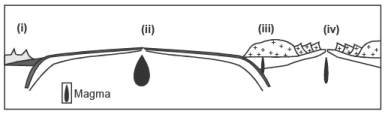Which one of the following options is correctly matched?

Solution:
QUESTION: 12

In an undulated topography, a set of uniformly inclined beds show ‘V’-shaped outcrop pattern with apex pointing downstream in a valley. This indicates:

Solution:
QUESTION: 13

Silica- rich viscous magmas often produce:

Solution:
QUESTION: 14

Identify the faults in the diagrams (i), (ii), and (iii)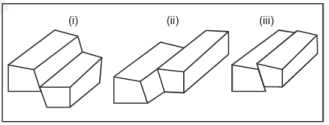Solution:
QUESTION: 15

A mine excavation made along the strike of a 2 meter thick tabular ore body dipping 30° is called:

Solution:
QUESTION: 16

An ore body showing ‘saddle reef’ structure is formed by:

Solution:
QUESTION: 17

In the given block diagram, select the most suitable site amongst (i), (ii), (iii), and (iv) for constructing a tunnel.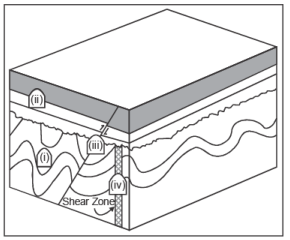Solution:
QUESTION: 18

Match the metals listed in Group- I with their ores in Group- II.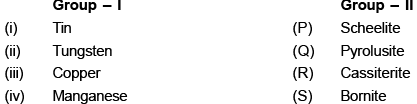Solution:
QUESTION: 19

Which one of the following minerals is correctly matched with its optical property?

Solution:
QUESTION: 20

The mineral coesite is expected to be stable in which of the following metamorphic facies?

Solution:
QUESTION: 21

In the given phase diagram, the sequence of minerals crystallizing from a magma of composition “X” under equilibrium conditions is: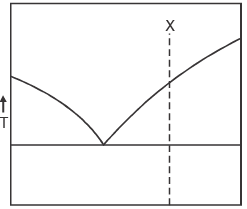Solution:
QUESTION: 22

In the given stereo plot, poles to three bedding planes are plotted as points (i), (ii) and (iii). Find the correct order of increasing dips of the beds.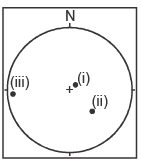Solution:
QUESTION: 23

Size of a kettle lake depends upon :

Solution:
QUESTION: 24

Match the sedimentary rocks given in Group- I with their characteristics in Group- II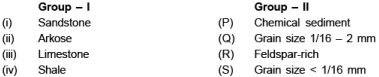Solution:
QUESTION: 25

Arrange the following formations of the Gondwana Supergroup in order of their decreasing age: (i) Raniganj Formation (ii) Barakar Formation (iii) Kulti Formation (iv) Karharbari Formation

Solution:
QUESTION: 26

Match the Lamellibranchia genera in Group- I with their dentition pattern in Group- II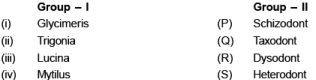Solution:
QUESTION: 27

Drainage density of a basin is defined as:

Solution:
QUESTION: 28

S- wave is terminated at:

Solution:
QUESTION: 29

Arrange the elements Fe, O, H, He, Si in decreasing order of their abundance in the solar system.

Solution:
QUESTION: 30

A dolerite dyke metamorphosed under amphibolite facies condition is expected to have the mineral assemblage:

Solution:
*Multiple options can be correct
QUESTION: 31

A pyrite- chalcopyrite- bearing copper ore body has undergone supergene enrichment. Choose the correct statement(s).

Solution:
*Multiple options can be correct
QUESTION: 32

The geochemical trends illustrated in the diagrams for a suite of cogenetic and coeval igneous rocks indicate :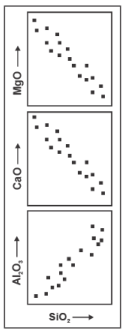Solution:
*Multiple options can be correct
QUESTION: 33

Which of the following chronostratigraphic unit(s) is/are correctly matched with the corresponding geochronologic unit(s) ?

Solution:
*Multiple options can be correct
QUESTION: 34

Which of the following statements related to depositional environments is/are correct ?

Solution:
*Multiple options can be correct
QUESTION: 35

W hich of the following fossil genera is/are invertebrate ?

Solution:
*Multiple options can be correct
QUESTION: 36

Which of the following trilobites have two to four thoracic segments and are eyeless?

Solution:
*Multiple options can be correct
QUESTION: 37

Which of the following formation(s) was/were deposited in glacial and/or fluvial environment?

Solution:
*Multiple options can be correct
QUESTION: 38

Which of the following statements is/are correct?

Solution:
*Multiple options can be correct
QUESTION: 39

Which of the following stratigraphic units are correctly matched with their ages and geographical localities?

Solution:
*Multiple options can be correct
QUESTION: 40

Petroleum producing basins of India belong to:

Solution:
*Answer can only contain numeric values
QUESTION: 41

On a toposheet of 1:50,000 scale, the distance between upper and lower traces of a bed is 5 cm. The actual distance on ground in kilometers is_________.

Solution:
*Answer can only contain numeric values
QUESTION: 42

On a flat topography the outcrop width of a bed is 30m. If the true dip of the bed is 30°, the actual thickness of the bed in meters is_________.

Solution:
*Answer can only contain numeric values
QUESTION: 43

In the given map, the true dip (in degree) of the bedding plane X- Y is_________.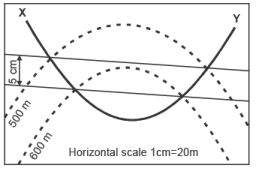Solution:
*Answer can only contain numeric values
QUESTION: 44

In the given diagram, the percentage of Plagioclase in a rock of composition ‘X’ is_________.

Solution:
*Answer can only contain numeric values
QUESTION: 45

The birefringence of a uniaxial mineral having refractive indices of 1.658 and 1.486 is_________.

Solution:
*Answer can only contain numeric values
QUESTION: 46

In the given stereoplot, the plunge amount of the fold axis is_________.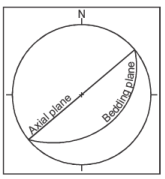Solution:
*Answer can only contain numeric values
QUESTION: 47

A crystal face has the following intercepts for the crystallographic axes: 1a1, 1a2, ½a3, ∞c. The Miller- Bravais indices for the face is_________.

Solution:
*Answer can only contain numeric values
QUESTION: 48

Total number of mirror planes in the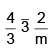point group is_________.

Solution:
*Answer can only contain numeric values
QUESTION: 49

A radioactive isotope has 1024 atoms. How many atoms will remain after 4 half- lives?

Solution:
*Answer can only contain numeric values
QUESTION: 50

Waste water discharged from a coal mine has hydrogen ion [H+] concentration of 10–6 moles/liter. The pH of the water is_________.

Solution:
*Answer can only contain numeric values
QUESTION: 51

An ore body is dipping 30° towards west on a flat topography. At what distance (in meters) should a borehole be placed so that it intersects the ore body perpendicularly at a vertical depth of 70 meters ?

Solution:
*Answer can only contain numeric values
QUESTION: 52

Using the phase rule, the maximum number of phases in a 3 (three) component geological system having 1 (one) degree of freedom is_________.

Solution:
*Answer can only contain numeric values
QUESTION: 53

The lithostatic pressure in Mega Pascal (MPa) at a depth of 10 km in a granite batholith having density 2700 kg/m3 is_________. (Acceleration due to gravity = 9.8 m/s2)

Solution:
*Answer can only contain numeric values
QUESTION: 54

In the given phase diagram, the weight percent of melt at point ‘M’ for a crystallizing magma of bulk composition ‘X’ is_________.

Solution:
*Answer can only contain numeric values
QUESTION: 55

Calculate the average atomic weight (answer to be given up to 3 decimal places) of Rubidium using the given data.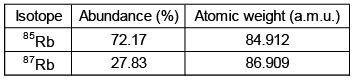Solution:
*Answer can only contain numeric values
QUESTION: 56

In an oblique slip fault having 30° dip, the net slip is 10 meters at an angle of 45° to the strike of the fault plane. The dip slip component of the fault in meters is_________. (Answer to be given up to 2 decimal places).

Solution:
*Answer can only contain numeric values
QUESTION: 57

A 10 cm3 sandstone block has a mass of 20 grams. If the average density of sand grains is 2.5 g/cm3, the porosity (in percent) of the sandstone is________.

Solution:
*Answer can only contain numeric values
QUESTION: 58

A mineral has a weight of 4.5 grams in air and 3.2 grams in water. Assuming density of water to be 1g/cm3, the specific gravity (up to 2 decimal places) of the mineral is_________.

Solution:
*Answer can only contain numeric values
QUESTION: 59

The duration of the Proterozoic Eon in Ma is_________.

Solution:
*Answer can only contain numeric values
QUESTION: 60

From the given equation, calculate how many moles of magnetite will be produced by the reduction of 1 (one) mole of hematite (answer to be given up to 3 decimal places). .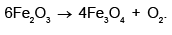Solution: